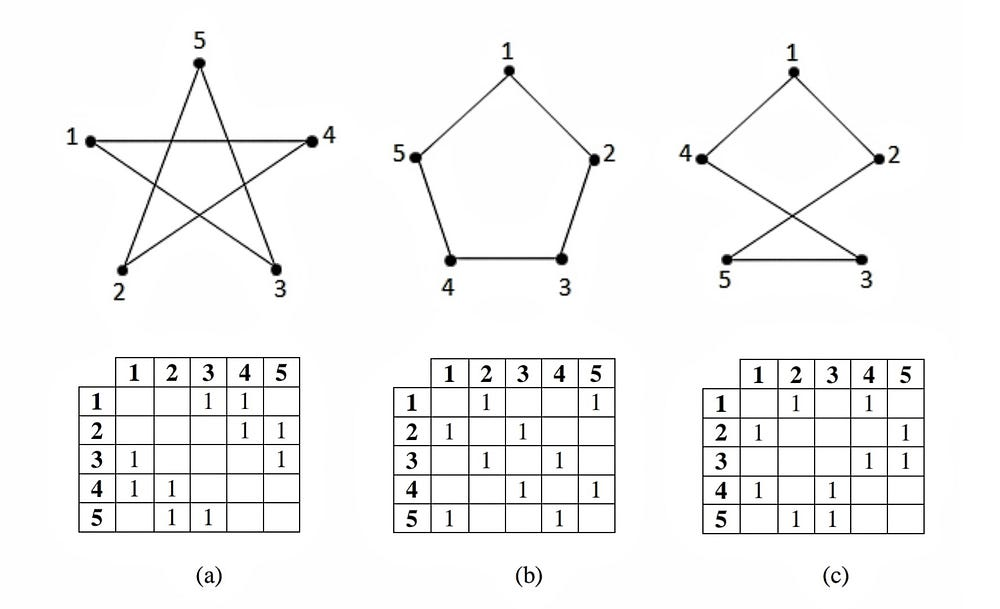# UnboxAI — Introduction to Graph Machine Learning

Original article was published on Deep Learning on MediumTL;DR Representing Graphs using Adjacency Matrix, Adjacency List, Incidence Matrix and node degree matrix

In this post, we will understand what is a graph, and different ways to represent it. Specifically

3. (Edge) Incidence Matrix
4. (Node) Diagonal Degree Matrix

# Introduction

A Graph is simply a collection of vertices/nodes and edges — where vertices typically represent entities and edges associate a relationship between the entities

Say for example — we have graph of Users (say Facebook/LinkedIN). Each user is a node in the graph. And all the people we are friends with (they will be nodes too!), will be represented using an edge to capture the friend-relationship.

Another example, lets take a graph of a road network — each node in the graph can represent a location (a pair of lat/lons) and edge between two locations describe the road or connectivity relationship.

As you might be already wondering now — hey these graphs have billions nodes and edges.. They are Huge!! Yes they are Big Graphs and by just by leveraging the network information we can extract meaningful relationships.

Lets take my LinkedIN profile (a node in the LinkedIN user Graph). I have connected with some profiles —they become my 1st degree connection. If there someone connected to “my connections” (but not to me) — they become my 2nd connection in the graph. This kind of reachability and propagation information in the graph is what populates your daily feeds, decide who will be an Influencer etc.

Ok — now that we can understand a node looks like me, and an edge looks like a friend-relationship etc. But how does a computer understand nodes and edges? This leads us to Graph Representation

# Graph Representation

There are 2 major ways to represent a graph